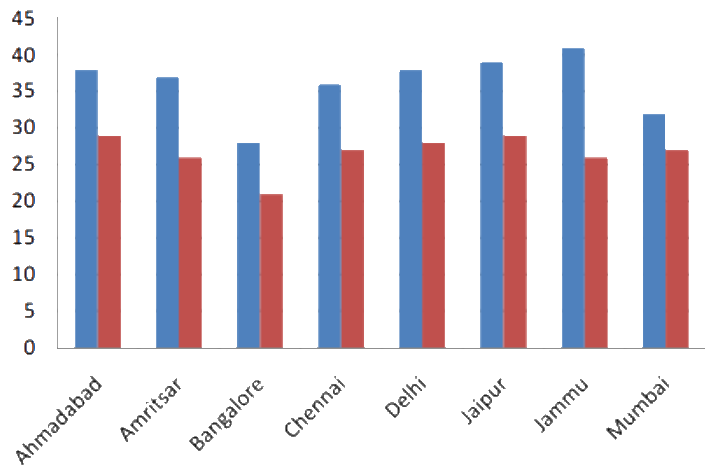# Ex.3.3 Q6 Data Handling - NCERT Maths Class 7

## Question

Take the data giving the maximum and minimum temperatures of various cities given in the beginning of this chapter (in the table below). Draw a double bar graph using the data and answer the following.

(i) Which city has the highest difference in the minimum and maximum temperature on the given date?

(ii) Which is the hottest city and which is the coldest city?

(iii) Name two cities where maximum temperature of one was less than the minimum temperature of the other.

(iv) Name the city which has the least difference between its minimum and maximum temperature?

Video Solution
Data Handling
Ex 3.3 | Question 6

## Text Solution

Steps:Temperature of cities as on $$20.06.2006$$

 City Maximum Minimum Ahmedabad $$38^\circ \rm C$$ $$29 ^\circ \rm C$$ Amritsar $$37 ^\circ \rm C$$ $$26 ^\circ \rm C$$ Bangalore $$28 ^\circ \rm C$$ $$21 ^\circ \rm C$$ Chennai $$36 ^\circ \rm C$$ $$27 ^\circ \rm C$$ Delhi $$38^\circ \rm C$$ $$28 ^\circ \rm C$$ Jaipur $$39 ^\circ \rm C$$ $$29 ^\circ \rm C$$ Jammu $$41 ^\circ \rm C$$ $$26 ^\circ \rm C$$ Mumbai $$32 ^\circ \rm C$$ $$27 ^\circ \rm C$$

Difference between the maximum and minimum temperature on $$20.06.2006$$

Ahmedabad $$= (38^\circ \rm C - 29^\circ \rm C) = 9^\circ \rm C$$

Amritsar $$=$$ ($$37 ^\circ \rm C$$ $$-$$ $$26^\circ \rm C$$) $$=$$ $$9 ^\circ \rm C$$

Bangalore $$=$$ ($$28^\circ \rm C$$ $$-$$ $$21^\circ \rm C$$) $$=$$ $$7 ^\circ \rm C$$

Chennai $$=$$ ($$36^\circ \rm C$$ $$-$$ $$27^\circ \rm C$$) $$=$$ $$9 ^\circ \rm C$$

Delhi $$=$$ ($$38 ^\circ \rm C$$ $$-$$ $$28^\circ \rm C$$) $$=$$ $$10 ^\circ \rm C$$

Jaipur $$=$$ ($$39 ^\circ \rm C$$ $$-$$ $$29 ^\circ \rm C$$) $$=$$ $$10 ^\circ \rm C$$

Jammu $$=$$ ($$41 ^\circ \rm C$$ $$-$$ $$26^\circ \rm C$$) $$=$$ $$15 ^\circ \rm C$$

Mumbai $$=$$ ($$32 ^\circ \rm C$$ $$-$$ $$27^\circ \rm C$$) $$=$$ $$5^\circ \rm C$$

(i)  As, it is clear from the above calculation, Jammu has the largest difference in the minimum and maximum temperature on the given data i.e. $$=$$ $$15 ^\circ \rm C$$.

(ii) Jammu is the hottest city with $$41^\circ \rm C$$ and Bangalore is the coldest city with $$21^\circ \rm C$$.

Bangalore and Jaipur or Bangalore and Ahmedabad are the two cities where maximum temperature of one was less than the minimum temperature of other.

Mumbai has the least difference between its minimum and maximum temperature.
Maximum temperature $$= 32^\circ \rm C$$
Minimum temperature $$=$$ $$27^\circ \rm C$$
∴ Difference $$=$$ ($$32 ^\circ \rm C$$ $$-$$ $$27^\circ \rm c$$) $$=$$ $$5^\circ \rm C$$

Learn from the best math teachers and top your exams

• Live one on one classroom and doubt clearing
• Practice worksheets in and after class for conceptual clarity
• Personalized curriculum to keep up with school